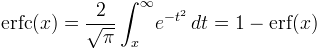Computes the complementary error function.

Namespace:  Meta.Numerics.Functions
Assembly:  Meta.Numerics (in Meta.Numerics.dll) Version: 4.1.4Syntax
```public static double Erfc(
double x
)```

#### Parameters

x
Type: SystemDouble
The argument.

#### Return Value

Type: Double
The value of erfc(x) = 1 - erf(x).Remarks

The complementary error function can be defined by an integral, or by its relation to the error function.The area in the tails of a bell curve ((NormalDistribution) beyond ∓z standard deviations from the mean is given by erfc(z/√2).

For small values of x, erfc(x) ≈ 1 to within floating-point accuracy. To obtain accurate values of erf(x) = 1 - erfc(x) in this region, use the Erf(Double) function.

The inverse of the complementary error function is available as InverseErfc(Double).

Values of the complementary error function for complex arguments can be obtained using the Faddeeva(Complex) function.See Also